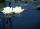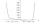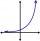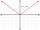Sum-log

The sum of two numbers is 32, the sum of their logarithms (base 10) is 2.2. Determine these numbers.

Result

x =  25.875
y =  6.125

Solution:Leave us a comment of example and its solution (i.e. if it is still somewhat unclear...):Be the first to comment!To solve this example are needed these knowledge from mathematics:

Looking for help with calculating roots of a quadratic equation? Do you have a linear equation or system of equations and looking for its solution? Or do you have quadratic equation?

Next similar examples:

1. Exponential equationSolve exponential equation (in real numbers): 98x-2=9
2. Water liliesWater lilies are growing on the pond and their number is doubled every day. The whole layer is covered in 12 days. How many days will it cover 8 layers?
3. Ball gameRichard, Denis and Denise together scored 932 goals. Denis scored 4 goals over Denise but Denis scored 24 goals less than Richard. Determine the number of goals for each player.
4. Unknown numberI think number. If subtract from the twelfth square the ninth square I get a number 27 times greater than the intended number. What is this unknown number?
5. Theorem proveWe want to prove the sentence: If the natural number n is divisible by six, then n is divisible by three. From what assumption we started?
6. DiscriminantDetermine the discriminant of the equation: ?
7. RootsDetermine the quadratic equation absolute coefficient q, that the equation has a real double root and the root x calculate: ?
8. EquationEquation ? has one root x1 = 8. Determine the coefficient b and the second root x2.
9. Intercept with axisF(x)=log(x+4)-2, what is the x intercept
10. Powers 32 to the power of n divided by 4 to the power of -3 equal 4. What is the vaule of n?
11. The city 3The city has 22,000 residents. How long it is expected to have 25,000 residents if the average annual population growth is 1.4%?Find the roots of the quadratic equation: 3x2-4x + (-4) = 0.Solve quadratic equation: (6n+1) (4n-1) = 3n2If 5x + x² > 100, then x is notOn the trip went men, women and children in the ratio 2:3:5 by bus. Children pay 60 crowns and adults 150. How many women were on the bus when a bus was paid 4,200 crowns?Calculate the roots of the equation: ?Solve two equations with two unknowns: 400x+120y=147.2 350x+200y=144